## 数学结构

### 上下标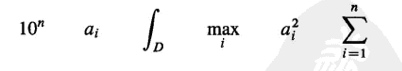$A_{ij} = 2^{i+j}$ $A_{ij} = 2^{i+j}$
$A = 90^\circ$ $A = 90^\circ$
$\int_0^1 f(t) \dif t = \iint_D g(x, y) \dif x \dif y$ $\int_0^1 f(t) \cos t = \iint_D g(x, y) \cos x \sin y$

### 上下画线与花括号

• \overline和\underline命令可用来在公式的上方和下方划横线
• \overbrace和\underbrace带上花括号

$\overline{a+b} = \overline a \overline b$ $\overline{a+b} = \overline a \overline b$
$\overbrace {a+b+c} = \underbrace{1+2+3}$ $\overbrace {a+b+c} = \underbrace{1+2+3}$

### 分式

$\frac{1}{\frac 12 (a+b)} = \frac{2}{a+b}$ $\frac{1}{\frac 12 (a+b)} = \frac{2}{a+b}$

### 根式

$\sqrt[n] {\frac{x^2 + \sqrt 2}{x+y}}$ $\sqrt[n] {\frac{x^2 + \sqrt 2}{x+y}}$

### 矩阵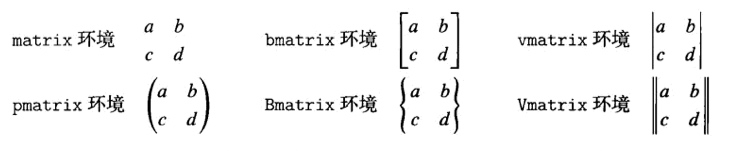$A = \begin{pmatrix} a & b & c \\ 0 & 1 & 0 \\ 0 & 0 & a \end{pmatrix}$ $A = \begin {pmatrix} a & b & c \\ 0 & 1 & 0 \\ 0 & 0 & a \end {pmatrix}$
$A = \begin{bmatrix} a_{11} & \dots & a_{1n} \\ & \ddots & \vdots \\ 0 & & a_{nn} \end{bmatrix}$ $A = \begin{bmatrix} a_{11} & \dots & a_{1n} \\ & \ddots & \vdots \\ 0 & & a_{nn} \end{bmatrix}$

## 符号与类型

### 字母表和普通符号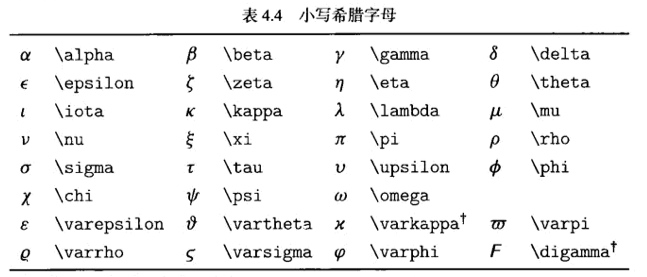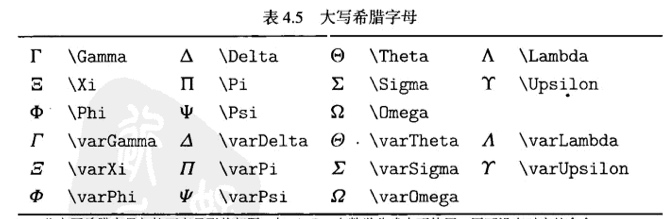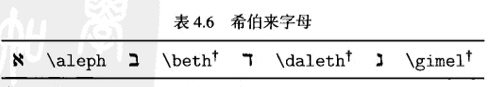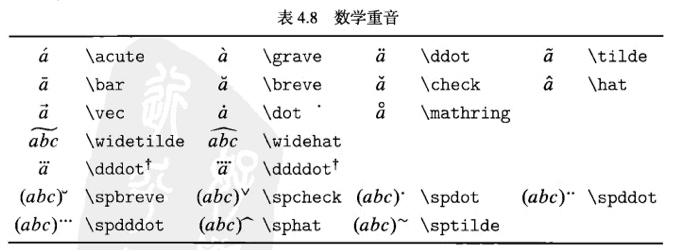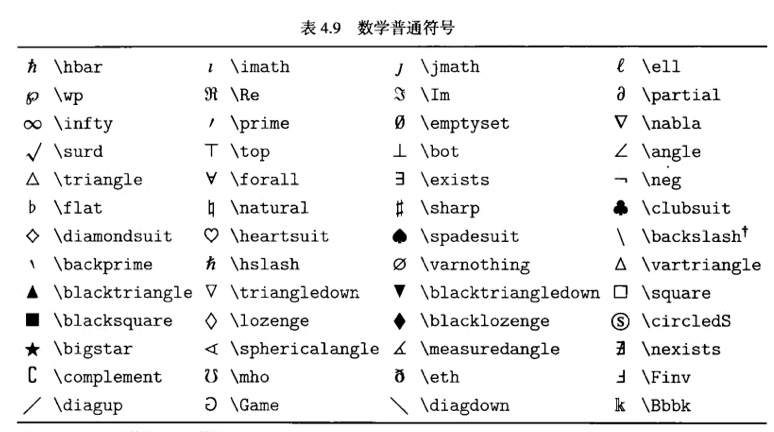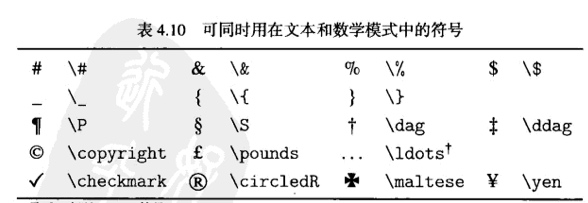### 数学算子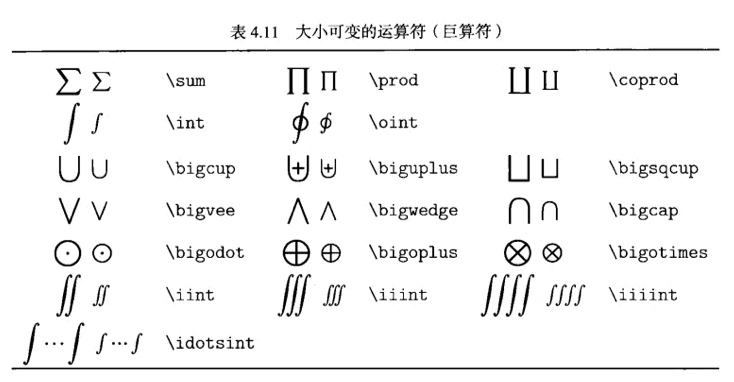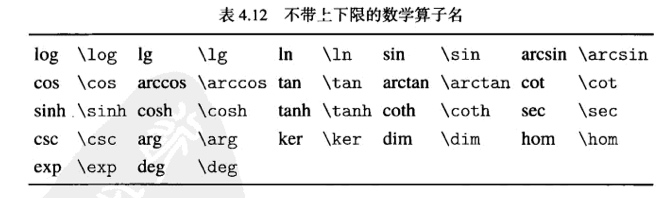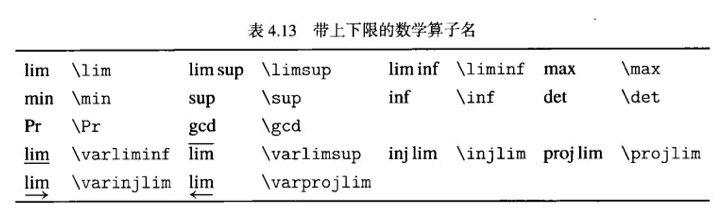### 二元运算符与关系符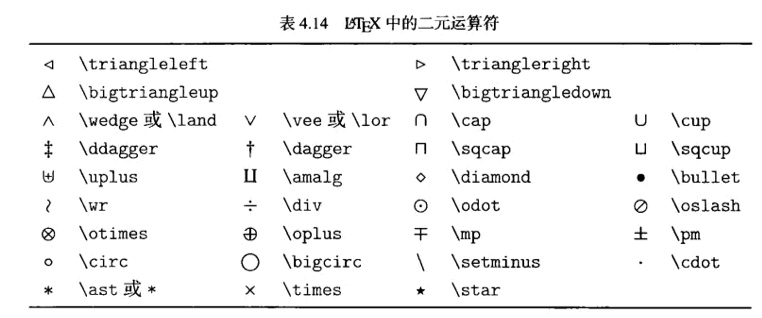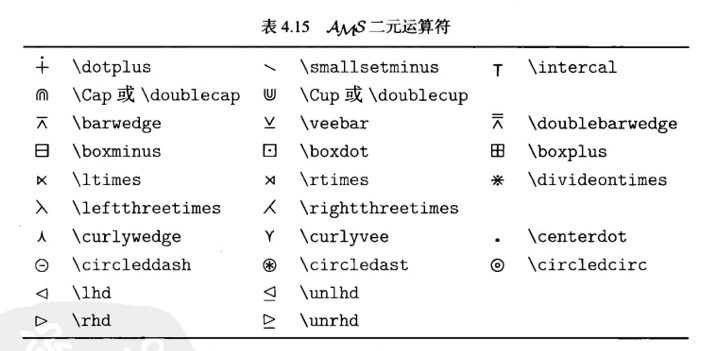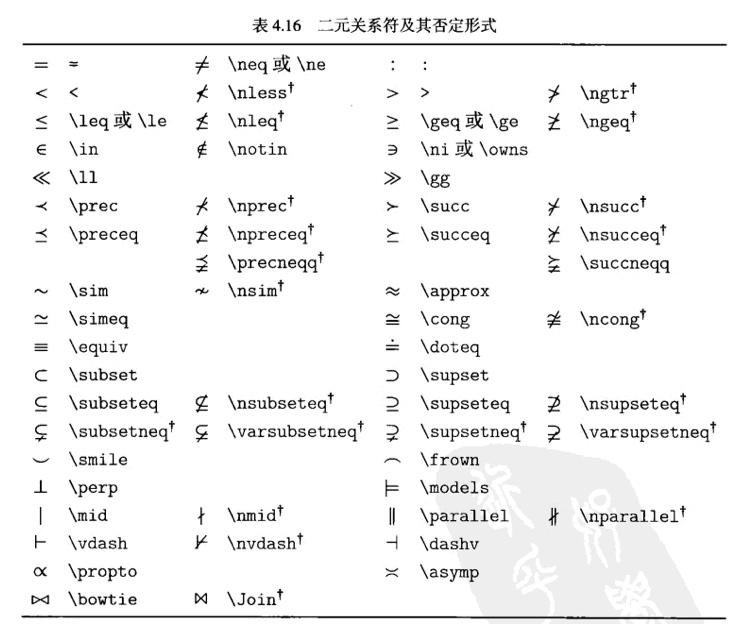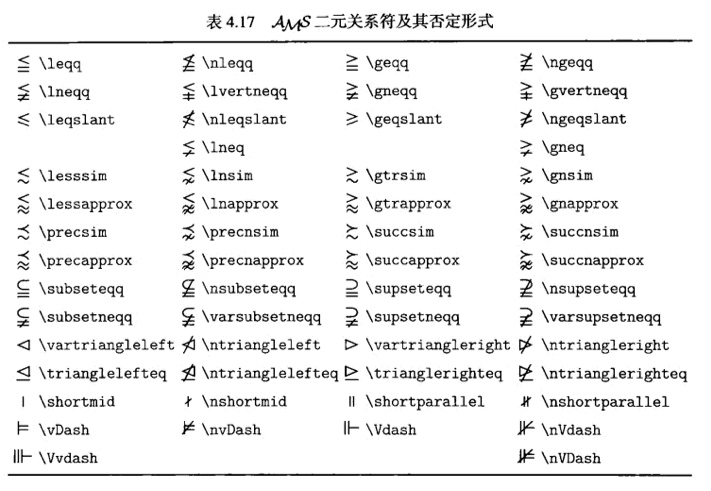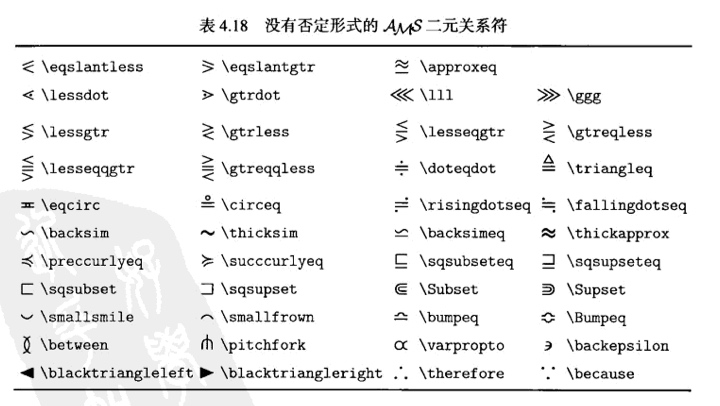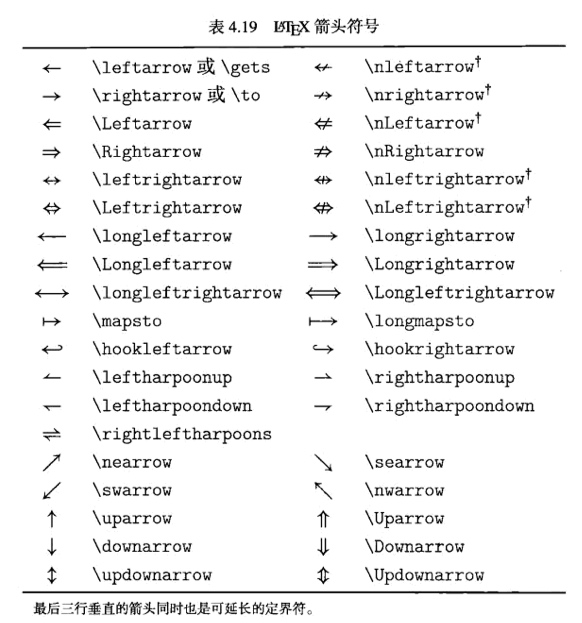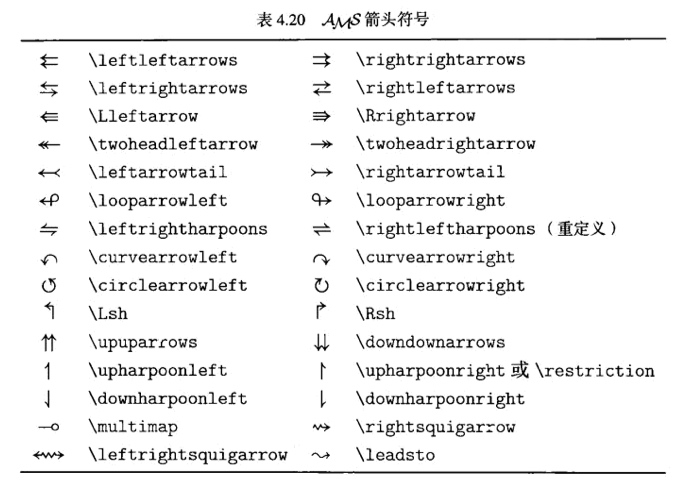### 括号与定界符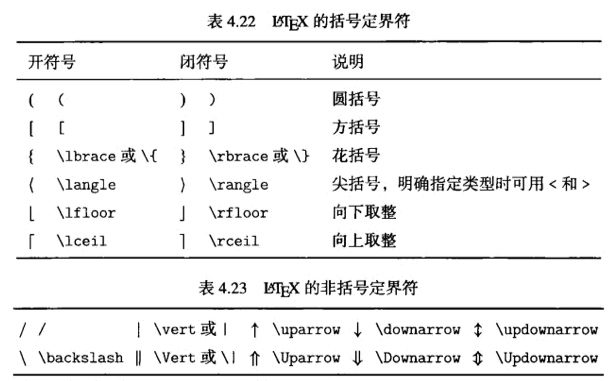### 标点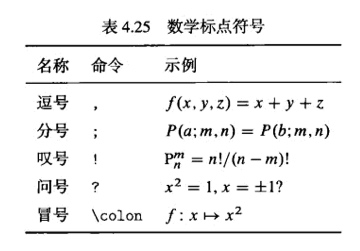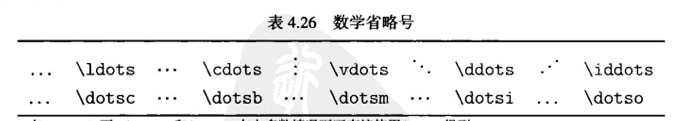## 在Hexo中使用LaTeX编辑数学公式

• 后面接, 各种括号的时候，前面_所表示符号前面要加\转义